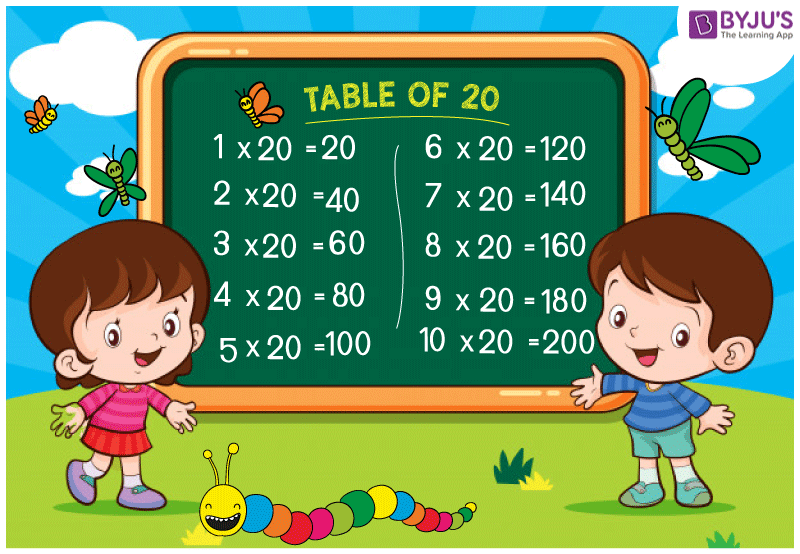# Table of 20

Table of 20 is essential for both schools going students and for students who are preparing for competitive exams, other national and international exams. Memorizing the table of 20 will help the students to solve complicated mathematical problems faster. To help students learn the table of 20 we have provides 20 times table here.## Multiplication Table of 20

 20 X 1 = 20 20 X 2 = 40 20 X 3 = 60 20 X 4 = 80 20 X 5 = 100 20 X 6 = 120 20 X 7 = 140 20 X 8 = 160 20 X 9 = 180 20 X 10 = 200 20 X 11 = 220 20 X 12 = 240 20 X 13 = 260 20 X 14 = 280 20 X 15 = 300 20 X 16 = 320 20 X 17 = 340 20 X 18 = 360 20 X 19 = 380 20 X 20 = 400

Visit BYJU’S for latest CBSE syllabus. We also provide CBSE notes for each and every class.

Get More Maths Tables:-

#### 1 Comment

1. AMIT KUMAR

VERY USEFUL FOR THE CHILD . THX